#### The exponential map

The steering problem will be solved by performing calculations on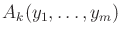. The formal power series of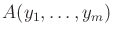is the set of all linear combinations of monomials, including those that have an infinite number of terms. Similarly, the formal Lie series of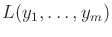can be defined.

The formal exponential map is defined for any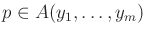as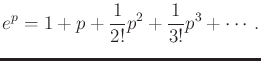(15.116)

In the nilpotent case, the formal exponential map is defined for any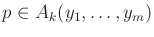as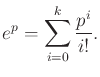(15.117)

The formal series is truncated because all terms with exponents larger thanvanish.

A formal Lie group is constructed as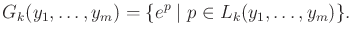(15.118)

If the formal Lie algebra is not nilpotent, then a formal Lie group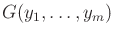can be defined as the set of all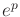, in which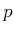is represented using a formal Lie series.

The following example is taken from :

Example 15..22 (Formal Lie Groups)   Suppose that the generatorsand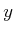are given. Some elements of the formal Lie group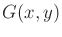are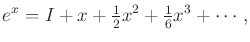(15.119)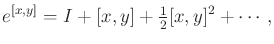(15.120)

and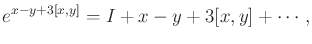(15.121)

in which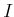is the formal Lie group identity. Some elements of the formal Lie group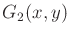are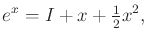(15.122)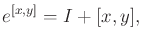(15.123)

and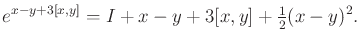(15.124)To be a group, the axioms given in Section 4.2.1 must be satisfied. The identity is, and associativity clearly follows from the series representations. Eachhas an inverse,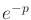, because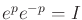. The only remaining axiom to satisfy is closure. This is given by the Campbell-Baker-Hausdorff-Dynkin formula (or CBHD formula), for which the first terms for any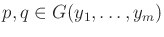are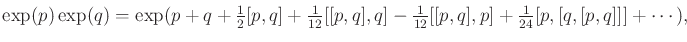(15.125)

in which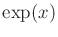alternatively denotes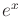for any. The formula also applies to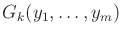, but it becomes truncated into a finite series. This fact will be utilized later. Note that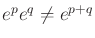, which differs from the standard definition of exponentiation.

The CBHD formula is often expressed as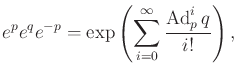(15.126)

in which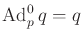, and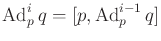. The operator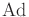provides a compact way to express some nested Lie bracket operations. Additional terms of (15.125) can be obtained using (15.126).

Steven M LaValle 2020-08-14